# Kerala Syllabus 8th Standard Maths Solutions Chapter 4 Identities

## Kerala State Syllabus 8th Standard Maths Solutions Chapter 4 Identities

### Identities Text Book Questions and Answers

Textbook Page No. 68

Identities Class 8 State Syllabus Question 1.
Write numbers as shown below.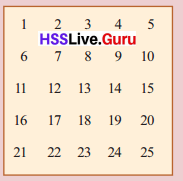(i) Mark a square of four numbers as shown in the calendar then find the difference by multiplying angle to angle. Is the difference same when 4 numbers from any of the square is taken?
(ii) Explain the reason by using algebra.
(iii) Instead of 4 numbers, take a square of nine numbers and mark the numbers from 4 corners.Solution:
(i)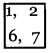6, 7
6 × 2 – 1 × 7
= 12 – 7 = 55 When 4 numbers of any square is taken we get the same difference.

(ii) If first number is x when we consider any of the square,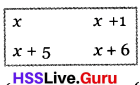we get in this form (x + 5) (x + 1) – x (x + 6)
(x2 + 6x + 5) – (x2 + 6x)
= x2 + 6x + 5 – x2 – 6x = 5
so any square is taken the difference is 5

(iii)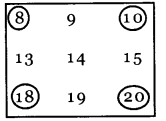Difference = 18 × 10 – 8 × 20 = 180 – 160 = 20
We can represent the numbers at the 4 corners from the square of nine numbers in the method of algebra as follows.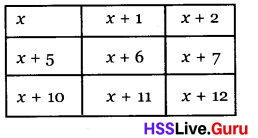Difference is
(x + 10) (x + 2) – x (x + 12)
(x2 + 12x + 20) – (x2 + 12x)
(x2 + 12x + 20 – x2 – 12 x) = 20
so, when any difference is taken we can find that the answer is 20.

Class 8 Maths Chapter 4 Identities Question 2.
Instead of 4 numbers take the square of nine numbers as shown in the previous table and mark the numbers at the four corners.(i) Find the difference of angle to angle sum?
(ii) Explain with the help of algebra, why we get the same difference in all such squares?
(iii) Explain the case of the square of 16 numbers?
Solution:
(i) (6 + 20) – (12 + 10)
= 26 – 22
= 4

(ii) If we write the square of 9 numbers in the algebraic method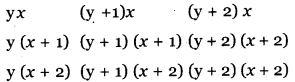Difference of angle to angle sum
[yx + (y + 2)(x + 2)] – [y(x + 2) + (y + 2)x]
[(yx + yx + 2x + 2y + 4) – ( yx + 2y + xy + 2x)] = 4

(iii) If we take the square of 16 numbers.
We get the form as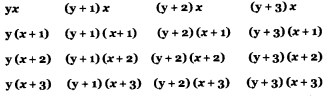The difference of the angle to angle sum is ,
[yx + (y + 3)(x + 3)] – [y(x + 3) + (y + 3)x]
[yx + yx + 3x + 3y + 9] – [yx + 3y + yx + 3x] = 9

Kerala Syllabus 8th Standard Maths Identities Question 3.
Observe the following operations
1 × 4 = (2 × 3) – 2
2 × 5 = (3 × 4) – 2
3 × 6 = (4 × 5) – 2
4 × 7 = (5 × 6) – 2
(i) Write next operations of two rows in the same order.
(ii) Among the 4 nearest natural numbers. Find the relation- ship between the product of first and last and those in the middle.
(iii) Write the principle in algebraic expression and explain the reason
Solution:
(i) 5 × 8 = (6 × 7) – 2
6 × 9 = (7 × 8) – 2
(ii) The product of first and last numbers = 2 subtracted from the product of two middle numbers.
(iii) The numbers are n, n+1, n+2, (n+3)
n (n+3) = n2 + 3n
(n + 1) (n + 2) = n2 + 3n + 2
difference = 2

Kerala Syllabus 8th Standard Maths Notes Question 4.
The method to find the product of 46 × 28 is shown below.(i) Observe the method in 2 two digit numbers.
(ii) Two digit numbers are expressed in the algebraic form, 10 m + n
Solution:
(i) 57 × 24(ii) If ab, cd are the numbers
ab × cd
(10a + b) (10c + d)
10a × 10c + 10a × d + 10c × b + b × d
100 × ac + 10 (ad + cb) + bd

Textbook Page No. 71

8th Class Maths Identities Question 1.
Find the squares of the given numbers by using simple calculations.
(i) 52
(ii) 105
(iii) 20$$\frac{1}{2}$$
(iv) 10.2
Solution:
(i) 522 = (50 + 2)2
= 502 + 2 × 50 × 2 + 22
= 2500 + 200 + 4
= 2704

(ii) 1052 = (100 + 5)2
= 1002 + 2 × 100 × 5 + 52
=10000 + 1000 + 25
= 11025

(iii) (20 $$\frac{1}{2}$$)2 =(20 + $$\frac{1}{2}$$)2
= 202 + 2 × 20 ×$$\frac{1}{2}$$ + ($$\frac{1}{2}$$)2
= 400 + 20 +$$\frac{1}{4}$$
= 420$$\frac{1}{4}$$

(iv) (10.2)2 = (10 + 0.2)2
= 102 + 2 × 10 × 0.2 + (0.2)2
= 100 + 4 + 0.04
= 104.04

Textbook Page No. 74

Hsslive Guru 8th Class Maths Question 1.
Is there any common method to find the squares of numbers like 1$$\frac{1}{2}$$, 2$$\frac{1}{2}$$, 3$$\frac{1}{2}$$ etc. Explain with the help of algebra?
Solution: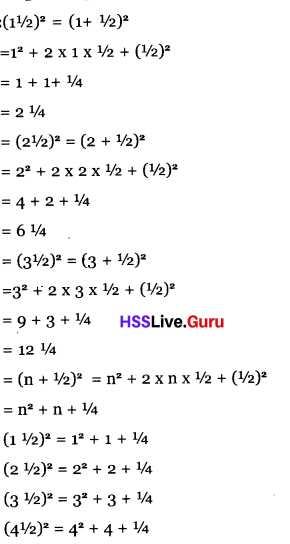We can adopt an easy method to find out the square of numbers like 1$$\frac{1}{2}$$, 2 $$\frac{1}{2}$$, 3$$\frac{1}{2}$$ as
(n + $$\frac{1}{2}$$)2 = n2 + n + $$\frac{1}{4}$$
Eg: (10 $$\frac{1}{2}$$)2 = 102 + 10 + $$\frac{1}{4}$$
= 100 + 10 + $$\frac{1}{4}$$
= 110$$\frac{1}{4}$$

Kerala Syllabus 8th Standard Notes Maths Question 2.
The method to find 372 is shown below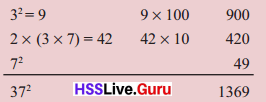(i) Use this method to find the square of other 2 digit numbers.
(ii) Explain algebraic method to get correct answer in this method.
(iii) Find an easy method to find the square of numbers end in 5
Solution:
(i) Square of 23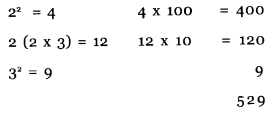(ii) Number = xy
10x + y
(10x + y)2
= (10x)2 + 2 × 10x × y + y2
= 100x2 + 10 (2 xy) + y2
= x2 x 100 + 2 x y × 10 + y2
= 100x2 + 20xy + y2

(iii) 10 (x + 5)2
= 102x2 + 2 × 10x × 5 + 52
= 100 x2 + 100 x + 25
= 100 (x2 + x) + 25
Eg:
152 = 100 (12 + 1) + 25
= 200 + 25= 225
252 = 100 (22 + 2) + 25
= 600 + 25 = 625
352 = 100 (32 + 3) + 25
= 1200 + 25 = 1225
Multiply the sum of first number and its square with 100 and add 25.

Hss Live Guru Class 8 Maths Question 3.
Observe the following expressions.
12 + (4 × 2) = 32
22 + (4 × 3) = 42
32 + (4 × 4) = 52
(i) Write two more expressions we get
(ii) What is the common principles from this? Explain on the basis of algebra
Solution:
(i) 42 + (4 × 5) = 62
52 + (4 × 6) = T
(ii) n2 + 4(n + 1) = (n + 2)2
n2 + 4n + 4 = (n + 2)2

8th Standard Maths Guide Kerala Syllabus Question 4.
Explain on the basis of algebra that any natural number which is not a multiple of 3 when divided by 3, we get the remainder as 1.
Solution:
We can write the natural numbers which are not the multiple of 3 as.
3n + 1, 3 n + 2
(3n + 1)2 = (3n)2 + 6n + 1
= 9n2 + 6n + 1
= 3 (3n2 + 2n) + 1
When divided by 3, remainder is 1
(3n + 2)2 = 9n2 + 6n + 4
= 9n2 + 6n + 3 + 1
= 3 (3n2 + 2n + 1) + 1
When divided by 3, remainder is 1

8th Standard State Syllabus Maths Solutions Question 5.
Prove that, the squares of numbers which end in 3 end in 9.
Solution:
We can write the numbers end in 3 as
10x + 3 is (10x + 3)2
=(10x)2 + 6ox + 9; So it ends in 9.

Textbook Page No. 79

8th Maths Solutions State Syllabus Question 1.
Find the squares of the numbers given below.
(i) 49
(ii) 98
(iii) 7 $$\frac{3}{4}$$
(iv) 9.25
Solution:
(i) 492 = (50 – 1)2
= 502 – 2 × 50 × 1 + 1
= 2500 – 100 + 1
= 2401

(ii) 982 = (100 – 2)2
= 1002 – 2 × 100 × 2 + 22
= 10000 – 400 + 4
= 9604

(iii) (7 $$\frac{3}{4}$$)2 = (8 – $$\frac{1}{4}$$)2
= 82 – 2 × 8 × $$\frac{1}{4}$$ + ($$\frac{1}{4}$$)2
= 64 – 4 + $$\frac{1}{16}$$
= 60$$\frac{1}{16}$$

(iv) 9.25 2
= (10 -.75)2
= 102 – 2 × 10 × .75 + (.75)2
= 100 – 15 + 0.5625
= 85. 5625

8th Standard Maths 4th Chapter Question 2.
Observe the followingExplain the common principle in these using algebra?
Solution:
(x – $$\frac{1}{2}$$)2 + (x + $$\frac{1}{2}$$)2
x2 – x + $$\frac{1}{4}$$ + x2 + x + $$\frac{1}{4}$$
2x2 + $$\frac{1}{2}$$

Question 3.
Some of the natural numbers can be written as the difference of two squares as.
242 = 72 – 52 = 52 – 12
32 = 92 – 72 = 62 – 22
40 = 112 – 92 = 72 – 32
(i) Explain the method to write the multiples of 8 from 24 onwards in this method on the basis of algebra.
(ii) In how many methods the multiples of 16 from 48 onwards to write the difference of perfect squares?
Solution:
(i) 4xy = (x + y)2 – (x – y)2
24 = 4 × 6 × 1 = ( 6 + 1)2 – (6 – 1)2
= 72 – 52
24 = 4 × 3 × 2 = (3 + 2)2 – (3 – 2)2
= 52 – 12
The multiples of 8 from 24 onwards can be written in two forms as 4 × x × y
Number = 4 × y = (r + y)2 – (r – y)2
Number = 4 ab = (a + b)2 – (a – b)2
32 = 4 × 8 × 1
= (8 + 1)2 – (8 – 1)2
= 92 – 72
32 = 4 × 4 × 2
= (4 + 2)2 – (4 – 2)2
= 62 – 22
40 = 4 × 10 × 1
= (10 + 1)2 – (10 – 1)2 = 112 – 92
40 = 4 × 5 × 2
= (5 + 2)2 – (5 – 2 )2 = 72 – 32

(ii) 48 = 4 × 4 × 3, 4 × 12 × 1, 4 × 6 × 2
There are the different ways of writing 48. So it can be written in 3 different methods as the difference of perfect squares.
48 = 4 × 4 × 3
= (4 + 3)2 – (4 – 3)2 = 72 – 12
48 = 4 × 12 × 1
= (12 + 1)2 – (12 – 1 )2 = 132 – 112
48 = 4 × 6 × 2
= (6 + 2)2 – (6 – 2)2 = 82 – 42

Textbook Page No. 81

Maths Notes For Class 8 State Syllabus Question 1.
Calculate the answers of the following questions by using mental calculations.
(i) (a) 682 – 32
(b) (31$$\frac{1}{2}$$)2 – (2$$\frac{1}{2}$$)2
(c) 3.62 – 1.42
(ii) (a) 201 × 99
(b) 2 $$\frac{1}{3}$$ × 1$$\frac{2}{3}$$
(c) 10.7 × 9.3
Solution:(c) 10.7 × 9.3
= (10 + 0.7) (10 – 0.7)
= 102 – 0.72
= 100 – 0.49
= 99.51

8th Standard Maths Identities Question 2.
Observe the following expressions.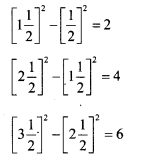Explain the common method in these using algebra.
Solution:
n2 – (n – 1)2
= n2 – (n2 – 2n+ 1)
= n2 – n2 + 2n – 1
= 2n – 1
n2 – (n – 1)2 = 2n – 1Kerala Syllabus 8th Standard Maths Guide Question 3.
Find, where we get big number among the following pairs without multiplying them?
(i) 25 × 75, 26 × 74
(ii) 76 × 24 . 74 × 26
(iii) 10.6 × 9.6
(iv) 10.4 × 9.6
Solution:
(i) 26 × 74
(ii) 74 × 26
(iii) 10.6 × 9.6
Indications
25 × 75 = (50 – 25) (50 + 25)
= 502 – 252
26 × 74 = (50 – 24) (50 + 24)
= 502 – 242

Class 8 Maths State Syllabus Question 4.
Find the difference in the given questions.
(i) (125 × 75) – (126 × 74)
(ii) (124 × 76) – (126 × 74)
(iii) (224 × 176) – (226 × 174)
(iv) (10.3 × 9.7) – (10.7 × 9.3)
(v) (11.3 × 10.7) – (11.7 × 11.3)
Solution:
(i) (125 × 75) – (126 × 74)
(100 + 25) (100 – 25) – (100 + 26) (100 – 26)
(1002 – 252) – (1002 – 262)
= 262 – 252
= (26 + 25) (26 – 25)
= 51

(ii) (124 × 76) – (126 × 74)
(100 + 24) (100 – 24) – (100 + 26) (100 – 26)
(1002 – 242) – (1002 – 26)2
= 262 – 242
= (26 + 24) (26 – 24)
= 50 × 2
= 100

(iii) (224 × 176) – (226 × 174)
(200 + 24) (200 – 24) – (200 + 26) (200 – 26)
(2002 – 242) – (2002 – 262)
= 262 – 242
= (26 + 24) (26 – 24)
= 50 × 2
= 100

(iv) (10.3 × 9.7) – (10.7 × 9.3)
(10 + 0.3) (10 – 0.3) – (10 + 0.7) (10 – 0.7)
(102 – 0.32) – (102 – 0.72)
= 0.72 – 0.32
= (0.7 + 0.3) (0.7 – 0.3)
= 1 × .4 = .4

(v) (11.3 × 10.7) – (11.7 × 11.3)
(11 + 0.3) (11 – 0.3) – (11 + 0.7) (11 – 0.7)
(112 – 0.32) – (112 – 0.72)
= 0.72 – 0.32
= (0.7 + 0.3) (0.7 – 0.3)
= 1 × 0.4 = 0.4

Textbook Page No. 85

State Syllabus 8th Class Maths Question 1.
4 numbers in a square is marked on the calendar.Add the squares of number pairs in angle to angle. Find out the difference of these sums?
42 + 122 = 160
112 + 52 = 146
160 – 146 = 14
(i) In the same was mark other 4 numbers and work out the questions.
(ii) Explain the reason on the basis of algebra that in all the squares we get the difference 14.
Solution:
(i)52 + 132 = 25 + 169 = 194
122 + 62 = 144 + 36 = 180
194 – 180 = 14

(ii) Let the first number in the square is x[x2 + (x + 8)2] – [(x + 7)2 + (x + 1)2]
[x2 + x2 + 16x + 64] – [x2 + 14x + 49 + x2 + 2x + 1]
= 14

Question 2.
Take nine numbers forming a sq¬uare in a calendar and mark the four numbers at the corners.Add the squares of diagonal pai¬rs and find the difference of the sums.
32 + 192 = 370
172 + 52 = 314
370 – 314 = 56
(i) Do this for other such nine numbers.
(ii) Explain using algebra, why the difference is always 56. (it is convenient to take the number at the centre of the square as x – see the section
Solution: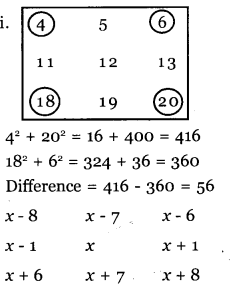(ii) If we represent a square with 9 nu-mbers
[(x – 8)2 +(x + 8)2] – [(x + 6)2 + (x – 6)2]
[x2 + 82 + x2 + 82] – [x2 + 62 + x2 + 62]
(82 + 82) – (62 + 62) = 128 – 72 = 56

Question 3.
Take a square of nine numbers in the calendar and make the 4 numbers in the 4 corners.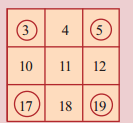Multiply the angle to angle number pairs. Find the difference of the products.
3 × 19 = 57
17 × 5 = 85
85 – 57 = 28
(i) Take a square of nine numbers and follow the same method as above.
(ii) Explain the reason why we get the difference in the^squares as 28 on the basis of algebra.
Solution:
(i)18 × 6 – 4 × 20
108 – 80 = 28

(ii)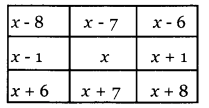(x + 6) (x – 6) – (x – 8) (x + 8)
(x2 – 62) – (x2 + 82)
= x2 – 62 – x2 – 82
= 82 – 62
= (8 + 6) (8 – 6)
= 14 × 2 = 28

Question 1.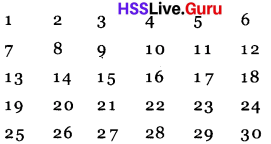(a) Take a square of 4 numbers and multiply angle to angle and find the difference. Is the difference the same when 4 numbers are taken from the comers in any square?
(b) Explain the reason by using the principle of algebra.
(c) Take a square of 9 numbers multiply angle to angle and find the difference. Explain on the basis of algebra.
Solution:
(a)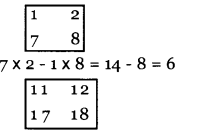17 × 12 – 11 × 18 = 204 – 198 = 6 We get the same difference.

(b) Take the square with the numbers as follows.(n + 6) ( n + 1) – n (n + 7)
(n2 + 7) (n + 6) – (n2 + 7n)
= 6

(c)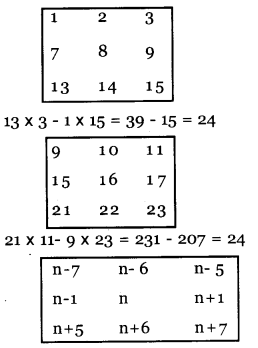(n + 5) (n – 5) – (n – 7) (n + 7)
(n2 – 52) (n2 – 72)
= 72 – 52
= 49 – 25 = 24

Question 2.
Find the square of 10.01
Solution:
10.012 = (10 + 0.01)2
= 102 + 2 × 10 × 0.01 + 0.012
= 100 + 0.2 + 0.0001 = 100.2001

Question 3.
Find the square of 99. 99
Solution:
99.992 = (100 – 0.01)2
= 1002 – 2 × 100 × 0.01 + 0.012
= 10000 – 2 + 0.0001 = 9998.0001

Question 4.
Find out the difference in the following question.
1. (18 × 12) – (20 × 10)
2. (131 × 19) – (135 × 17)
3. (222 × 78) – (224 × 76)
4. (12.4 × 2.6) – (12. 6 × 2.4)
Solution:
Use the same method of the 4th question in page 83 of the text book.